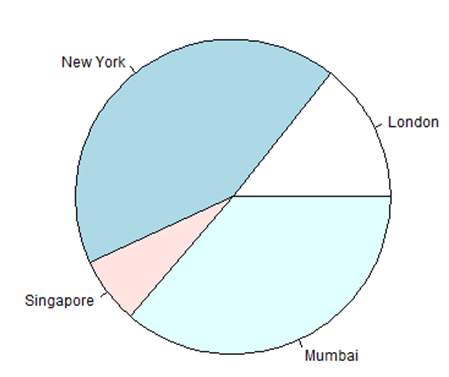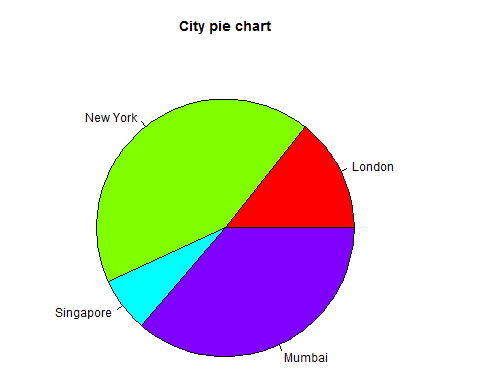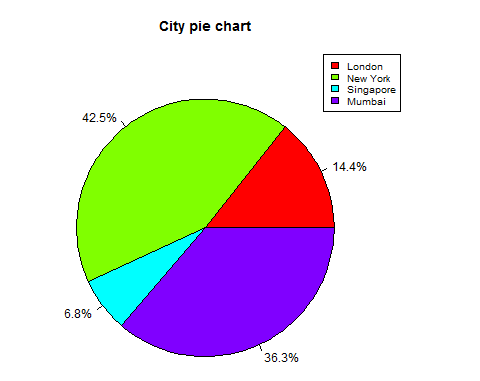### R在线运行

`极速运行模式，更高效的运行，点击编辑器上方的运行按钮即刻体验吧。`

# R语言 饼状图

R编程语言有许多库来创建图表和图表。 饼图是将值表示为具有不同颜色的圆的切片。 切片被标记，并且对应于每个片的数字也在图表中表示。

## 语法

``````pie(x, labels, radius, main, col, clockwise)
``````

• x是包含饼图中使用的数值的向量。

• labels用于给出切片的描述。

• main表示图表的标题。

• col表示调色板。

• clockwise是指示片段是顺时针还是逆时针绘制的逻辑值。

#### 例

``````# Create data for the graph.
x <- c(21, 62, 10, 53)
labels <- c("London", "New York", "Singapore", "Mumbai")

# Give the chart file a name.
png(file = "city.jpg")

# Plot the chart.
pie(x,labels)

# Save the file.
dev.off()
``````### 饼图标题和颜色

``````# Create data for the graph.
x <- c(21, 62, 10, 53)
labels <- c("London", "New York", "Singapore", "Mumbai")

# Give the chart file a name.
png(file = "city_title_colours.jpg")

# Plot the chart with title and rainbow color pallet.
pie(x, labels, main = "City pie chart", col = rainbow(length(x)))

# Save the file.
dev.off()
``````### 切片百分比和图表图例

``````# Create data for the graph.
x <-  c(21, 62, 10,53)
labels <-  c("London","New York","Singapore","Mumbai")

piepercent<- round(100*x/sum(x), 1)

# Give the chart file a name.
png(file = "city_percentage_legends.jpg")

# Plot the chart.
pie(x, labels = piepercent, main = "City pie chart",col = rainbow(length(x)))
legend("topright", c("London","New York","Singapore","Mumbai"), cex = 0.8,
fill = rainbow(length(x)))

# Save the file.
dev.off()
``````### 3D饼图

``````# Get the library.
library(plotrix)

# Create data for the graph.
x <-  c(21, 62, 10,53)
lbl <-  c("London","New York","Singapore","Mumbai")

# Give the chart file a name.
png(file = "3d_pie_chart.jpg")

# Plot the chart.
pie3D(x,labels = lbl,explode = 0.1, main = "Pie Chart of Countries ")

# Save the file.
dev.off()
``````JSRUN提供的R在线运行，R 在线运行工具，基于linux操作系统环境提供线上编译和线上运行，具有运行快速，运行结果与常用开发、生产环境保持一致的特点。

• 00:23